# 5.3 Galvanic Cells

Learning Objectives

By the end of this section, you will be able to:

• Describe the function of a galvanic cell and its components
• Use cell notation to symbolize the composition and construction of galvanic cells

As demonstration of spontaneous chemical change, (Figure 5.3.1) shows the result of immersing a coiled wire of copper into an aqueous solution of silver nitrate. A gradual but visually impressive change spontaneously occurs as the initially colorless solution becomes increasingly blue, and the initially smooth copper wire becomes covered with a porous gray solid.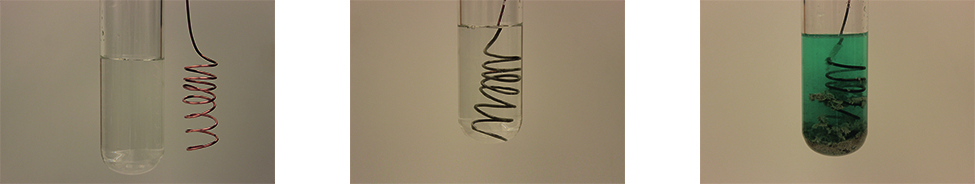Figure 5.3.1 – A copper wire and an aqueous solution of silver nitrate (left) are brought into contact (center) and a spontaneous transfer of electrons occurs, creating blue Cu2+(aq) and gray Ag(s) (right).

These observations are consistent with (i) the oxidation of elemental copper to yield copper(II) ions, Cu2+(aq), which impart a blue color to the solution, and (ii) the reduction of silver(I) ions to yield elemental silver, which deposits as a fluffy solid on the copper wire surface. And so, the direct transfer of electrons from the copper wire to the aqueous silver ions is spontaneous under the employed conditions. A summary of this redox system is provided by these equations: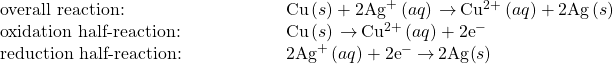Consider the construction of a device that contains all the reactants and products of a redox system like the one here, but prevents physical contact between the reactants. Direct transfer of electrons is, therefore, prevented; transfer, instead, takes place indirectly through an external circuit that contacts the separated reactants. Devices of this sort are generally referred to as electrochemical cells, and those in which a spontaneous redox reaction takes place are called galvanic cells (or voltaic cells).

A galvanic cell based on the spontaneous reaction between copper and silver(I) is depicted in (Figure 5.3.2). The cell is comprised of two half-cells, each containing the redox conjugate pair (“couple”) of a single reactant. The half-cell shown at the left contains the Cu(0)/Cu(II) couple in the form of a solid copper foil and an aqueous solution of copper nitrate. The right half-cell contains the Ag(I)/Ag(0) couple as solid silver foil and an aqueous silver nitrate solution. An external circuit is connected to each half-cell at its solid foil, meaning the Cu and Ag foil each function as an electrode. By definition, the anode of an electrochemical cell is the electrode at which oxidation occurs (in this case, the Cu foil) and the cathode is the electrode where reduction occurs (the Ag foil). The redox reactions in a galvanic cell occur only at the interface between each half-cell’s reaction mixture and its electrode. To keep the reactants separate while maintaining charge-balance, the two half-cell solutions are connected by a tube filled with inert electrolyte solution called a salt bridge. The spontaneous reaction in this cell produces Cu2+ cations in the anode half-cell and consumes Ag+ ions in the cathode half-cell, resulting in a compensatory flow of inert ions from the salt bridge that maintains charge balance. Increasing concentrations of Cu2+ in the anode half-cell are balanced by an influx of NO3 from the salt bridge, while a flow of Na+ into the cathode half-cell compensates for the decreasing Ag+ concentration.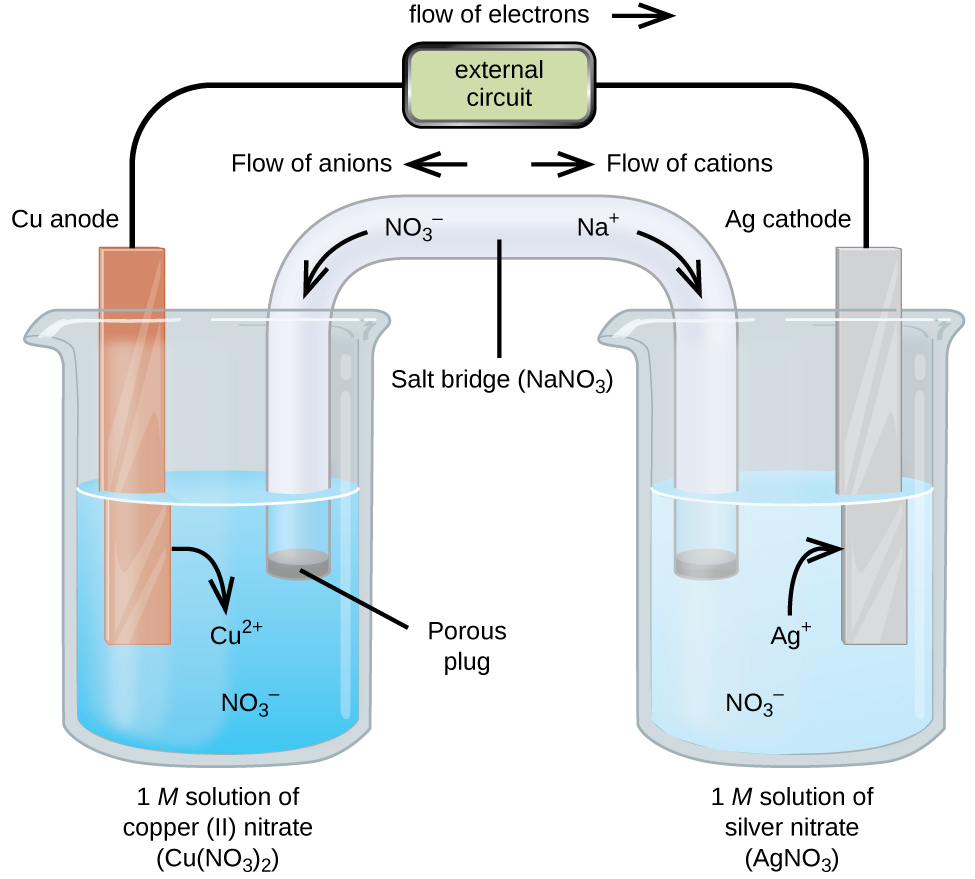Figure 5.3.2 – A galvanic cell based on the spontaneous reaction between copper and silver(I) ions.

## Cell Notation

Abbreviated symbolism is commonly used to represent a galvanic cell by providing essential information on its composition and structure. These symbolic representations are called cell notations or cell schematics, and they are written following a few guidelines:

• The relevant components of each half-cell are represented by their chemical formulas or element symbols
• All interfaces between component phases are represented by vertical parallel lines; if two or more components are present in the same phase, their formulas are separated by commas
• By convention, the schematic begins with the anode and proceeds left-to-right identifying phases and interfaces encountered within the cell, ending with the cathode

A verbal description of the cell as viewed from anode-to-cathode is often a useful first-step in writing its schematic. For example, the galvanic cell shown in (Figure 5.3.2) consists of a solid copper anode immersed in an aqueous solution of copper(II) nitrate that is connected via a salt bridge to an aqueous silver(I) nitrate solution, immersed in which is a solid silver cathode. Converting this statement to symbolism following the above guidelines results in the cell schematic: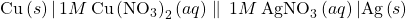Consider a different galvanic cell (see (Figure 5.3.3)) based on the spontaneous reaction between solid magnesium and aqueous iron(III) ions: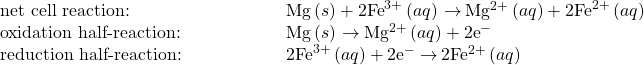In this cell, a solid magnesium anode is immersed in an aqueous solution of magnesium chloride that is connected via a salt bridge to an aqueous solution containing a mixture of iron(III) chloride and iron(II) chloride, immersed in which is a platinum cathode. The cell schematic is then written as: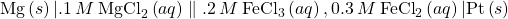Notice the cathode half-cell is different from the others considered thus far in that its electrode is comprised of a substance (Pt) that is neither a reactant nor a product of the cell reaction. This is required when neither member of the half-cell’s redox couple can reasonably function as an electrode, which must be electrically conductive and in a phase separate from the half-cell solution. In this case, both members of the redox couple are solute species, and so Pt is used as an inert electrode that can simply provide or accept electrons to redox species in solution. Electrodes constructed from a member of the redox couple, such as the Mg anode in this cell, are called active electrodes.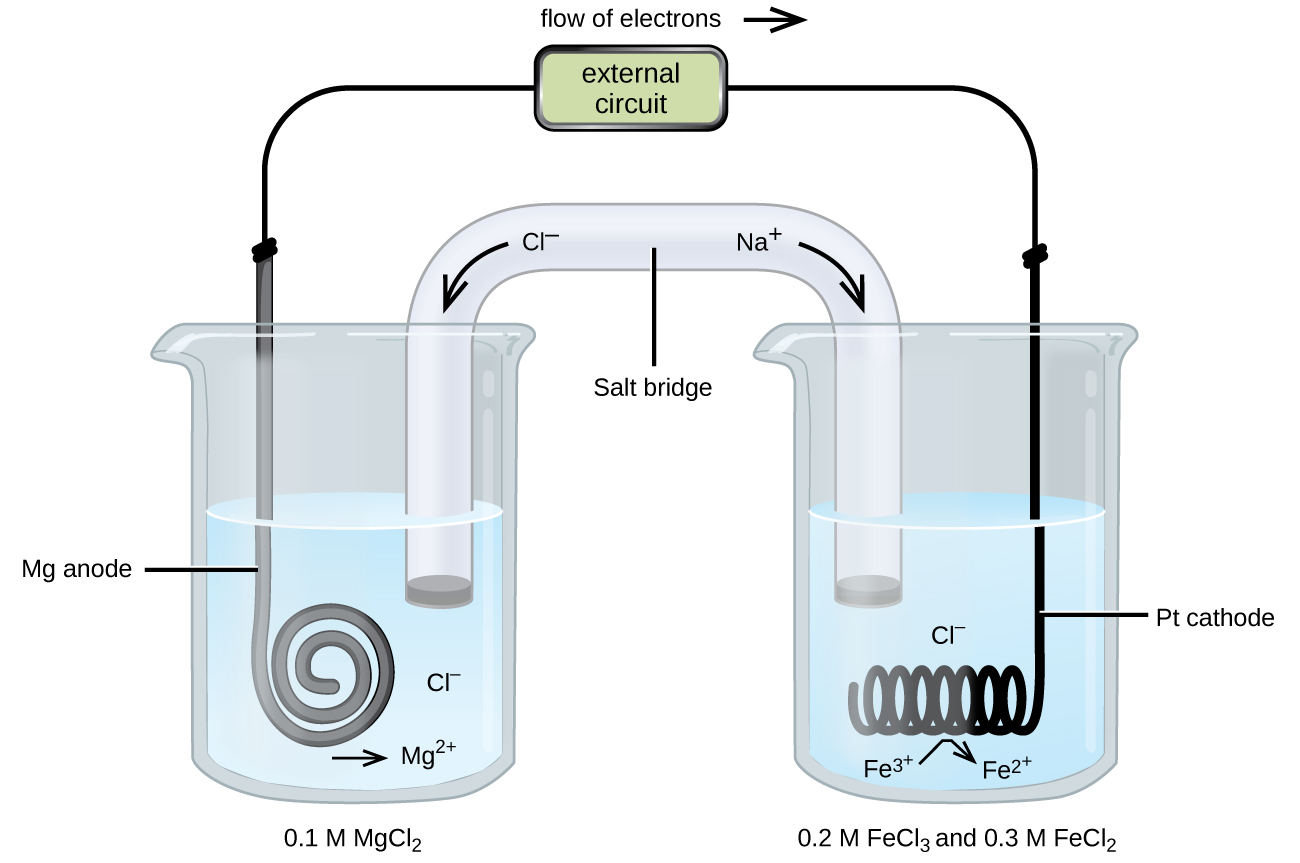Figure 5.3.3 – A galvanic cell based on the spontaneous reaction between magnesium and iron(III) ions.

Activity 5.3.1 – Writing Galvanic Cell Schematics

A galvanic cell is fabricated by connecting two half-cells with a salt bridge, one in which a chromium wire is immersed in a 1 M CrCl3 solution and another in which a copper wire is immersed in 1 M CuCl2. Assuming the chromium wire functions as an anode, write the schematic for this cell along with equations for the anode half-reaction, the cathode half-reaction, and the overall cell reaction.

## Solution

Since the chromium wire is stipulated to be the anode, the schematic begins with it and proceeds left-to-right, symbolizing the other cell components until ending with the copper wire cathode: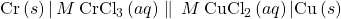The half-reactions for this cell are: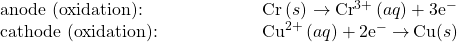Multiplying to make the number of electrons lost by Cr and gained by Cu2+ equal yields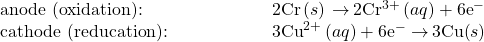Adding the half-reaction equations and simplifying yields an equation for the cell reaction: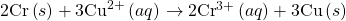Omitting solute concentrations and spectator ion identities, write the schematic for a galvanic cell whose net cell reaction is shown below: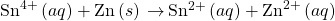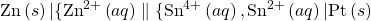# Key Concepts and Summary

Galvanic cells are devices in which a spontaneous redox reaction occurs indirectly, with the oxidant and reductant redox couples contained in separate half-cells. Electrons are transferred from the reductant (in the anode half-cell) to the oxidant (in the cathode half-cell) through an external circuit, and inert solution phase ions are transferred between half-cells, through a salt bridge, to maintain charge neutrality. The construction and composition of a galvanic cell may be succinctly represented using chemical formulas and others symbols in the form of a cell schematic (cell notation).

End of Chapter Exercises

(1) Write cell schematics for the following cell reactions, using platinum as an inert electrode as needed.

(1a)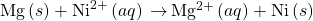(1b)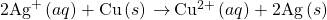(1c)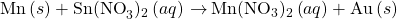(1d)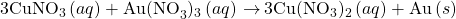Solution

(a)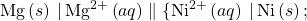(b)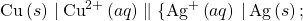(c)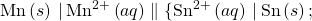(d)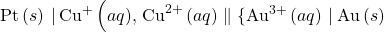(2) Assuming the schematics below represent galvanic cells as written, identify the half-cell reactions occurring in each.

(2a)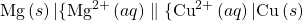(2b)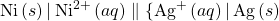(3) Write a balanced equation for the cell reaction of each cell in the previous exercise.

Solution

(a)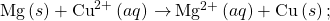(b)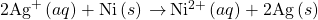(4) Balance each reaction below, and write a cell schematic representing the reaction as it would occur in a galvanic cell.

(4a)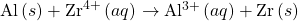(4b)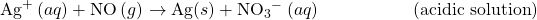(4c)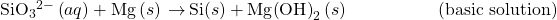(4d)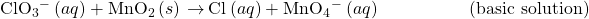(5) Identify the oxidant and reductant in each reaction of the previous exercise.

Solution

Species oxidized = reducing agent: (a) Al(s); (b) NO(g); (c) Mg(s); and (d) MnO2(s); Species reduced = oxidizing agent: (a) Zr4+(aq); (b) Ag+(aq); (c)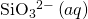; and (d)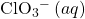(6) From the information provided, use cell notation to describe the following systems:

(6a) In one half-cell, a solution of Pt(NO3)2 forms Pt metal, while in the other half-cell, Cu metal goes into a Cu(NO3)2 solution with all solute concentrations 1 M.

(6b) The cathode consists of a gold electrode in a 0.55 M Au(NO3)3 solution and the anode is a magnesium electrode in 0.75 M Mg(NO3)2 solution.

(6c) One half-cell consists of a silver electrode in a 1 M AgNO3 solution, and in the other half-cell, a copper electrode in 1 M Cu(NO3)2 is oxidized.

(7) Why is a salt bridge necessary in galvanic cells like the one in (Figure 5.3.2)?

Solution

Without the salt bridge, the circuit would be open (or broken) and no current could flow. With a salt bridge, each half-cell remains electrically neutral and current can flow through the circuit.

(8) An active (metal) electrode was found to gain mass as the oxidation-reduction reaction was allowed to proceed. Was the electrode an anode or a cathode? Explain.

(9) An active (metal) electrode was found to lose mass as the oxidation-reduction reaction was allowed to proceed. Was the electrode an anode or a cathode? Explain.

Solution

Active electrodes participate in the oxidation-reduction reaction. Since metals form cations, the electrode would lose mass if metal atoms in the electrode were to oxidize and go into solution. Oxidation occurs at the anode.

(10) The masses of three electrodes (A, B, and C), each from three different galvanic cells, were measured before and after the cells were allowed to pass current for a while. The mass of electrode A increased, that of electrode B was unchanged, and that of electrode C decreased. Identify each electrode as active or inert, and note (if possible) whether it functioned as anode or cathode.

## Glossary

electrode that participates as a reactant or product in the oxidation-reduction reaction of an electrochemical cell; the mass of an active electrode changes during the oxidation-reduction reaction
electrode in an electrochemical cell at which oxidation occurs

electrode in an electrochemical cell at which reduction occurs

symbolic representation of the components and reactions in an electrochemical cell
(Ecell)
difference in potential of the cathode and anode half-cells
electrochemical cell in which a spontaneous redox reaction takes place; also called a voltaic cell
electrode that conducts electrons to and from the reactants in a half-cell but that is not itself oxidized or reduced